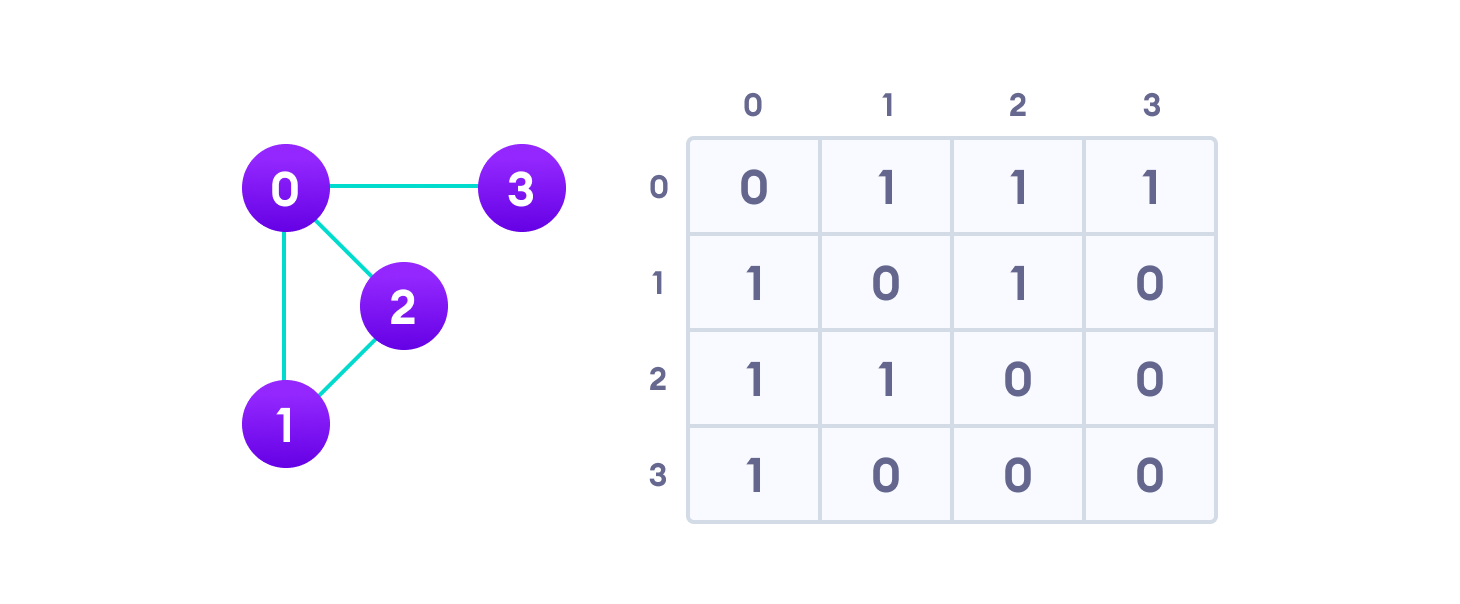Hits: 5

# Adjacency Matrix

#### In this tutorial, you will learn what an adjacency matrix is. Also, you will find working examples of adjacency matrix in Python.

An adjacency matrix is a way of representing a graph G = {V, E} as a matrix of booleans.

## Adjacency matrix representation

The size of the matrix is `VxV` where `V` is the number of vertices in the graph and the value of an entry `Aij` is either 1 or 0 depending on whether there is an edge from vertex i to vertex j.

## Adjacency Matrix Example

The image below shows a graph and its equivalent adjacency matrix.Adjacency matrix from a graph

In case of undirected graphs, the matrix is symmetric about the diagonal because of every edge `(i,j)`, there is also an edge `(j,i)`.

## Pros of adjacency matrix

The basic operations like adding an edge, removing an edge and checking whether there is an edge from vertex i to vertex j are extremely time efficient, constant time operations.

If the graph is dense and the number of edges is large, adjacency matrix should be the first choice. Even if the graph and the adjacency matrix is sparse, we can represent it using data structures for sparse matrices.

The biggest advantage however, comes from the use of matrices. The recent advances in hardware enable us to perform even expensive matrix operations on the GPU.

By performing operations on the adjacent matrix, we can get important insights into the nature of the graph and the relationship between its vertices.

## Cons of adjacency matrix

The `VxV` space requirement of the adjacency matrix makes it a memory hog. Graphs out in the wild usually don’t have too many connections and this is the major reason why adjacency lists are the better choice for most tasks.

While basic operations are easy, operations like `inEdges` and `outEdges` are expensive when using the adjacency matrix representation.

## Python Examples

If you know how to create two dimensional arrays, you also know how to create an adjacency matrix.

``````/* Adjacency Matrix representation in Python */

class Graph(object):

/* Initialize the matrix */
def __init__(self, size):
self.adjMatrix = []
for i in range(size):
self.adjMatrix.append([0 for i in range(size)])
self.size = size

/* Add edges */
def add_edge(self, v1, v2):
if v1 == v2:
print("Same vertex %d and %d" % (v1, v2))
self.adjMatrix[v1][v2] = 1
self.adjMatrix[v2][v1] = 1

/* Remove edges */
def remove_edge(self, v1, v2):
if self.adjMatrix[v1][v2] == 0:
print("No edge between %d and %d" % (v1, v2))
return
self.adjMatrix[v1][v2] = 0
self.adjMatrix[v2][v1] = 0

def __len__(self):
return self.size

/* Print the matrix */
def print_matrix(self):
for row in self.adjMatrix:
for val in row:
print('{:4}'.format(val)),
print

def main():
g = Graph(5)
g.add_edge(0, 1)
g.add_edge(0, 2)
g.add_edge(1, 2)
g.add_edge(2, 0)
g.add_edge(2, 3)

g.print_matrix()

if __name__ == '__main__':
main()``````

## Adjacency Matrix Applications

1. Creating routing table in networks
2. Navigation tasks

# Special 95% discount

## 2000+ Applied Machine Learning & Data Science Recipes

### Portfolio Projects for Aspiring Data Scientists: Tabular Text & Image Data Analytics as well as Time Series Forecasting in Python & R## Two Machine Learning Fields

There are two sides to machine learning:

• Practical Machine Learning:This is about querying databases, cleaning data, writing scripts to transform data and gluing algorithm and libraries together and writing custom code to squeeze reliable answers from data to satisfy difficult and ill defined questions. It’s the mess of reality.
• Theoretical Machine Learning: This is about math and abstraction and idealized scenarios and limits and beauty and informing what is possible. It is a whole lot neater and cleaner and removed from the mess of reality.

Data Science Resources: Data Science Recipes and Applied Machine Learning Recipes

Introduction to Applied Machine Learning & Data Science for Beginners, Business Analysts, Students, Researchers and Freelancers with Python & R Codes @ Western Australian Center for Applied Machine Learning & Data Science (WACAMLDS) !!!

Latest end-to-end Learn by Coding Recipes in Project-Based Learning:

Applied Statistics with R for Beginners and Business Professionals

Data Science and Machine Learning Projects in Python: Tabular Data Analytics

Data Science and Machine Learning Projects in R: Tabular Data Analytics

Python Machine Learning & Data Science Recipes: Learn by Coding

R Machine Learning & Data Science Recipes: Learn by Coding

Comparing Different Machine Learning Algorithms in Python for Classification (FREE)

`Disclaimer: The information and code presented within this recipe/tutorial is only for educational and coaching purposes for beginners and developers. Anyone can practice and apply the recipe/tutorial presented here, but the reader is taking full responsibility for his/her actions. The author (content curator) of this recipe (code / program) has made every effort to ensure the accuracy of the information was correct at time of publication. The author (content curator) does not assume and hereby disclaims any liability to any party for any loss, damage, or disruption caused by errors or omissions, whether such errors or omissions result from accident, negligence, or any other cause. The information presented here could also be found in public knowledge domains.  `